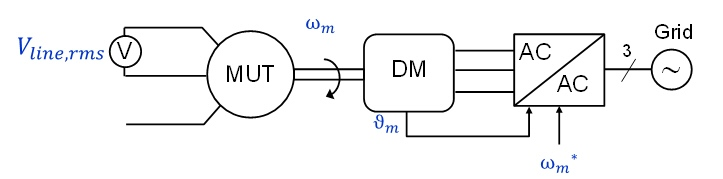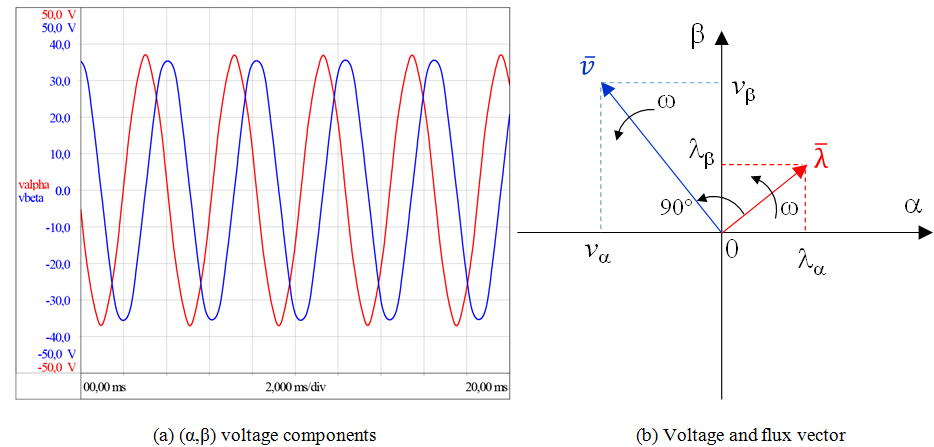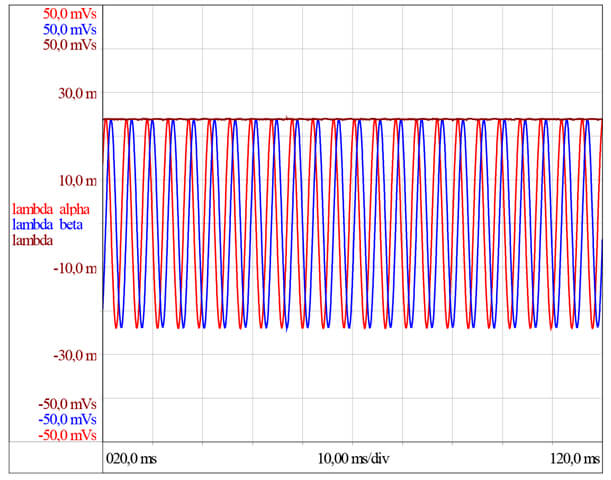Getting the Back-Emf Voltage Constant of Permanent Magnet Motors with Just One Twist of the Wrist using Gen3i Data Recorder

The parameter identification of permanent magnet motors is needed when a new motor prototype must be quickly analyzed. The parameter identification can be performed with complicated tests using inverter and a driving machine, or using simpler methods that do not need any inverter supply and prime movers. This paper focuses on a fast identification of the motor back-emf voltage constant using the HBM Gen3i data recorder. The motor is rotated by hand with just one twist of the wrist and the method is able to get very good results even for non-sinusoidal motors.

Due to their high torque density and higher efficiency with respect to other motor types, Permanent Magnet (PM) motors are able to create energy savings in a number of applications. The advancement of Adjustable Speed Drives (ASD) has caused PM motors to have expansion in many fields. Some of these fields include: traction, automotive, renewable power generation, electrical mobility, compressors electric aircraft and home appliances . DifferentPM machine designs are adopted to fulfil the application requirements. The rotor design determines the topology of the PM machine. The most common PM machines are Surface Mount (SM) PM machines, Interior Permanent Magnet (IPM) machines (single layer and multilayer rotors), inset PM machines, flux-concentrating PM machines and so on.

The application engineers who must implement specific motor control strategies need parameter identification procedures to get the motor parameters. The motor parameters that are usually used in motor control are: stator resistance, stator inductances and the magnets flux linkage (or the back-emf constant). The stator resistance and inductance can be obtained very quickly with line-to-line impedance measurement for different rotor positions or through a short-circuit test. The magnets flux is usually obtained by means of a no-load test (hereinafter called as conventional method); the Motor Under Test (MUT) is rotated by a Driving Motor (DM). The magnets flux is calculated from the induced voltage at the machine terminals and the electrical speed.

This paper proposes a very simple method for the identification of the PM motors magnets flux using the HBM Gen3i data recorder. Respect to the conventional method, the proposed method does not need a DM and it can be applied to PM motors having non-sinusoidal back-emf voltages. The paper is organized as follows. First, the conventional method is described in Section II. Then, the proposed method is analyzed in Section III. Section IV concludes the paper.

Conventional no-load test for back-emf constant identification

For the conventional no-load test, the MUT is driven at constant speed by a DM  with speed regulation, as shown in Fig.1.Fig. 1: Conventional method setup.

Assuming sinusoidal induced voltages at the MUT terminals, the rms line-to-line voltage is measured, as well as the motor speed ωm. Then the magnets flux is computed as:(1)

where p is the MUT pole-pairs number.

This method is simple but it has the following disadvantages:

• If the motor is wound to get non-sinusoidal back-emf voltages, then the calculated magnets flux is not correct since it must correspond only to the fundamental components
• If the speed is not constant (non ideal speed regulation and/or eccentricity of the MUT rotor shaft), the voltages will fluctuate

The non-ideal measurement conditions can be overcome by using the Gen3i data recorder, as described in Fig. 2.Fig. 2: Implementation of the conventional method using the HBM Gen3i dara recorder.

The data recorder directly measures the phase voltages, respect to the MUT neutral point. If the neutral point is not available, then the line-to-line voltages are measured instead. When the MUT is rotated at constant speed, the Gen3i acquires all of the data points from many electrical cycles, as shown in Fig. 3.Fig. 3: A long frame of data (about 2s) containing the motor voltage (left) and a zoom on these voltages (right).

As can be easily noted from Fig. 3, the induced voltagesare not sinusoidal, so the simple measurement of the rms value would produce an error in magnets flux computation. To properly compute the magnets flux, the space vectors theory is employed. First, the three-phase voltages (va,vb,vc) are transformed as bi-phase quantities in stationary (α,β) frame:(2)

The (α,β) voltage components are shown in Fig. 4a. These voltages are the time derivatives of the (α,β) flux components. Therefore, the flux components are obtained by the Gen3i using simple integration as:(3)

The (α,β) voltage and flux components are describing a back-emf vector and a flux vector that are orthogonal and rotate in the (α,β) plane with an angular frequency ω equal to the electrical frequency, as shown in Fig. 4b.Fig. 4: Voltage and flux vectors in (α,β) plane.

The voltage integration is simple and straightforward to get the flux components. However, any offset in the voltage measurement may cause flux drifting. In addition, according to the initial integration point, the fluxes may have nonzero mean values, as shown in Fig. 5. The Gen3i is able to detect properly each electrical cycle for the (α,β) voltages. If the mean value of the fluxes (calculated for each cycle) is subtracted from the integration result (Fig. 5-right), then the fluxes become ac quantities with zero mean values, as shown in Fig. 6.

Excepting the initial period of the time frame, the (α,β) flux components are near sinusoidal quantities. The magnets flux linkage that must be obtained is the mean value of the flux vector magnitude (see Fig.4b):(4)

The time interval during which the mean value is calculated must be properly chosen from the acquired frame.Fig. 5: Voltage integration results (left) and correction calculated for each cycle (right).

The final (α,β) flux components and the flux vector magnitude are shown in Fig. 7. The final value of the magnets flux has been obtained as

λm= 23.866 (mVs)   (5)Fig. 6: Flux component correction (left) and final (α,β) flux components (right).Fig. 7: Final (α,β) flux components and magnets flux as the magnitude of the flux vector.

Proposed method

Since the integration does not depend on the motor speed, it should be valid for any speed value, even if the speed is not constant. For this reason, the proposed method does not need a prime mover to rotate the MUT. The Gen3i records one long frame of voltage data while the MUT is rotated by hand with just one turn, as shown in Fig. 8. The phase and the (α,β) voltages are shown in Fig. 9.Fig. 8: Proposed method. The MUT is rotated by hand while the Gen3i acquires the induced voltages at the MUT terminals.Fig. 9: A long frame of data containing the MUT phase voltages (left) and the computed (α,β) voltage components (right).Fig. 10: Flux component correction (left) and final (α,β) flux components (right).

The voltage integration and the correction of the flux components are performed as explained in the previous section. The calculated magnets flux with this method is:

λm= 23.865 (mVs)   (6)

As can be easily noted, the calculated magnets flux is practically the same as the one computed using the conventional no-load test, demonstrating that the DM is not necessary.

Conclusions

The quick identification of a PM motor prototype requires several motor parameters that are required by the motor control. This paper focuses on the identification of the magnets flux linkage (back-emf constant). The conventional no-load test requires a driving machine that must rotate the MUT at constant speed. The proposed method does not need a driving motor and gest the motor flux after rotating the MUT rotor by hand with just one turn. The HBM data recorder easily gets the motor fluxes with voltage integration and proper correction of offsets in the integration process.

Authors

• R. Bojoi
• E. Armando

Politecnico di Torino, Dipartimento Energia
Corso Duca degli Abruzzi 24, 10129, Torino, Italy

Related Products

Power Analyzers
HBM eDrive testing consists of two components – the power analyzer and DAQ system which are used to test electrical drives.
GEN3i - Data Recorder with 3 Slots
GEN3i is a powerful and fast transient and data recorder. It offers both continuous direct-to-disk with up to 200 MB/s as well as fast transient recording.